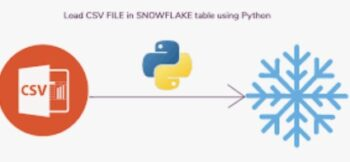As we know in pandas Data frame, to_Sql method is used to create or replace the table inside the Snowflake Database via the Python to Snowflake Connector. The to_sql method uses insert statements to insert the data into the table but with one limitation. It sends one line of values per row in the data frame which is fine for smaller Datasets. The same method errored out or causes the memory issue if running big resources aka huge dataset.

When you try to write a large pandas Data Frame with the `to_sql` method it converts the entire data frame into a list of values. During this transformation more RAM is utilizes than the original Data Frame. Moreover old Data Frame still remains present in RAM.

A way to solve this is to provide the `to_sql` method a chunksize argument during the data read from the Pandas library in below way:

`````` for prw in pd.read_sql_query('SELECT * FROM table, chunksize=100000):
prw .to_sql('table_name', con=ms_engine, index=false)``````

#### Chunksize Limitation:

But the above approach also not feasible due to `chunksize `limitation:

1) Using `chunksize` does not necessarily fetches the data from the database into python in chunks. By default it will fetch all data into memory at once, and only returns the data in chunks (so the conversion to a data frame happens in chunks).

2) Python does not necessarily free all memory that is not used anymore to the OS, so it will also depend on how you measured the memory usage (as you will probably know since you tried the garbage collector.

So we have come up with the alternative approach, Use a header-only Data Frame, via .head(0) to force the creation of an empty table.

Once the empty table gets created, we will leverage the functionality of Snowflake COPY command to perform the BULK load which will insert the data in one go instead of Row-By-Row as in case of to_sql method.

Now consider the requirement. Instead of getting the file from external system, we need to manipulate or ALTER the Table data and definition inside the Snowflake database.

#### Approach:

Python to Snowflake : Getting data from Snowflake database in pandas Data frame. Manipulating data inside the Data frame according to the business logic and finally ingest the transformed data into Snowflake.

• Addition of new columns in Data frame based on conditions.
• Grouping the data and Sum using transform function.
• Replace null values in DF column with desired value.
• Convert the Model field to upper case.
• Calculating the difference between dates in data frame
• Save the contents of the Data Frame to a file.
• Upload the file to internal location of machine
• Run a copy command in Snowflake to load the data.
``````import os
import pandas as pd
import sqlalchemy
import snowflake.connector
from snowflake.sqlalchemy import URL
from sqlalchemy import create_engine
import io
engine = create_engine(URL(
account='xxxxxxxx,
user='sachinsnowpro',
warehouse='COMPUTE_WH',
database='DEMO_DB',
schema='PUBLIC',
role='xxxxxx'
))
connection = engine.connect()
sql = "select * from policy_info";
for c in df.columns:
if c.endswith("_date"):
df[c] = pd.to_datetime(df[c])
df['status']=['Active' if x > 1000 else 'Inactive' for x in df['policy_amount']]
df['Policy days Difference'] = (df['policy_exp_date'] - df['policy_start_date']).dt.daysdf['opt/left']=['opt' if x > 0 else 'left' for x in df['policy_amount']]
df['Legacy Source'] = 'Policy'
df["Policy Account Total"] = df.groupby('policy_account')["policy_amount"].transform('sum')
##The tricky part is figuring out how to combine this data back with the original dataframe. .
##Using the original data, let’s try using transform and groupby and see what we get:
df["model"].fillna("Xerox", inplace = True)
df['model'] = df['model'].str.upper()
engine,
table_name,
create=True):
file_name = f"{table_name}.csv"
file_path = os.path.abspath(file_name)
with engine.connect() as con:
if create:
con=con,
if_exists="replace",
index=False)
con.execute(f"put file://{file_path}* @%{table_name}")
con.execute(f"copy into {table_name}")5 Star
0%
4 Star
0%
3 Star
0%
2 Star
0%
1 Star
0%